## bookmark_borderQuantifying Evidence (2): Evidence Is Limited By How Much a Study Can Be Trusted

In part 1, we defined evidence and showed that evidence across independent studies can be aggregated by addition; if Alice’s results provide 2 units of evidence and Bob’s results provide 3 units of evidence then we have a total of 5 units of evidence. The problem with this is that it doesn’t account for our intuition that single experiments cannot be trusted too much until they are replicated. 10 congruent studies each reporting 2 units of evidence should prevail over one conflicting study showing -20 units of evidence.

Let’s try to model this by assuming that every experiment has a chance of being flawed due to some mistake or systematic error. Each study can have its own probability of failure, in which case the results of that experiment should not be used at all. This is our first assumption: that any result is either completely valid or completely invalid. It is a simplification but a useful one.

We define trust (T) in a particular study as the logarithm of the odds ratio for the being valid versus being invalid. In formal terms: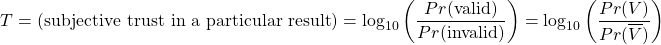A trust T=2 corresponds to a belief that the odds the outcome being flawed is 1 to 100. T=3 corresponds to an odds of 1 to 1000. In my view, 1<T<3 is reasonable for the typical study. But trust is something subjective that cannot be objectively calculated. Much like priors, it depends on the person sitting outside of the study interpreting its results.

Take a study with data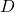that reports evidence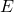. That study can either be valid or invalid (represented by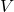and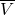). The reported evidence was calculated under the assumption that the study was valid. So: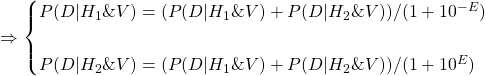From the perspective of an observer interpreting a study with trust, we can calculate the effective evidence,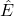.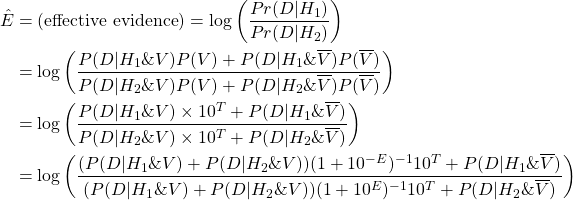We define G as the resulting evidence in case the study is invalid.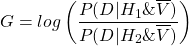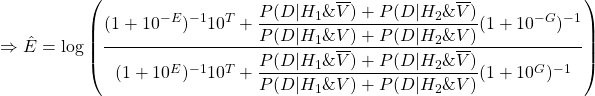Here we are going to make another simplification: G = 0. The second assumption is that if a study is invalid, it provides no evidence for or against H1 versus H2. This means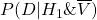=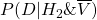=. Substituting for these values we get:And to simplify the above formula we define another term: believability. Believability (B) is defined below.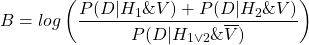Substituting B we get the following:It’s alright if you didn’t closely follow the math up to here. What is important is that we now have a formula for calculating effective evidence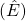based on reported evidence, trust, and believability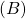.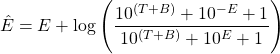The reported evidenceis an objective number we get from the study. Trustis a subjective quantity that the subjects interpreting the study must determine for themselves, independent of the outcome of the study. Believability is a bit more complex. Believability is a number ascribed to a particular outcome or observation, much like evidence is. But in contrast to evidence, believability cannot be determined objectively. This is because of the term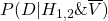which has to be determined by the interpreter; it is subjective and can vary for different people. I will write more about believability in the next part of this series. (Suffice it to say that a study can be designed to guarantee a believability of B≥0).

To gain a better understanding about how the above formula works, I made the following plot.

Effective evidence begins to grow linearly with respect to reported evidence. But it plateaus at (T+B). In other words, evidence is effectively limited by how much a study can be trusted plus the believability of the study’s outcome. To first approximation, the magnitude of effective evidence is roughly equal to min(|E|, T+B). This approximation is least accurate when |E| T+B or when T+B < 1.

This formalizes our intuition that no single study can be used to decisively confirm or deny a hypothesis, no matter how strong the evidence turns out to be in that study. The amount of trust one places in a study limits the amount of evidence that can be acquired from it. For example, if you place a trust of T=1.5 in the typical paper, no single study can convince you by more than 1.5 units of evidence (assuming B=0; more on believability later). You would need to add the effective evidence () from multiple independent studies to establish that there is higher than 1.5 units of evidence for something. This aspect of our framework is nice, because astronomically large or small values are commonplace when working with likelihood ratios. But by accounting for trust, extremely large amounts of reported evidence are not extremely informative.

A meta analysis of multiple studies can be done by calculating the effective evidence for each study and then summing the values. 10 studies that each report 2 units of evidence will almost certainly prevail over one [conflicting] study that reports -20 units of evidence, given that no study can reasonably be trusted with T≥20. If T = 3 and B = 0, then the overall evidence in this case is is 10×2-3 = 9. (Each of the first 10 studies will have an effective evidence of 2 and the single conflicting study will have an effective evidence of -3).

Now, here is a problem that will lead us to the next part. How do we deal with believability? From the perspective of a researcher, we would like to minimize it since it effectively limits the evidence that can be deduced from a study.

If the outcome of an experiment is a continuous value, then all the probabilities in the above formulas become marginal probabilities, meaning that the denominator can get infinitesimally small. The numerator depends on the person evaluating our study and can be infinitely large for some inconveniently skeptical interpreter. So there is no limit to how negative believability can get! If believability is not dealt with in a study, there is no guarantee that an interpreter will take away any information from that study. What can be done to guarantee something like this will not happen? I will discuss this in part 3.

## bookmark_borderQuantifying Evidence (1): What Are Units of Evidence?

I am going to introduce a statistical framework for quantifying evidence as a series of blog posts. My hope is that by doing it through this format, people will understand it, build on these ideas, and actually use it as a practical replacement for p-value testing. If you haven’t already seen my post on why standard statistical methods that use p-values are flawed, you can check it out through this link.

My proposal builds on Bayesian hypothesis testing. Bayesian hypothesis testing makes use of the Bayes factor, which is the likelihood ratio of observing some data D for two competing hypotheses H1 and H2. A Bayes factor larger than 1 counts as evidence in favor of hypothesis H1; a smaller than one Bayes factor counts as evidence in favor of H2.

In classical hypothesis testing, we typically set a threshold for the p-value (say, p<0.01) below which a hypothesis can be rejected. But in the Bayesian framework, no such threshold can be defined as hypothesis rejection/confirmation will depend on the prior probabilities. Prior probabilities (i.e., the probabilities assigned prior to seeing data) are subjective. One person may assign equal probabilities for H1 and H2. Another may think H1 is ten times more likely than H2. And neither can be said to be objectively correct. But the Bayesian method leaves this subjective part out of the equation, allowing anyone to multiply the Bayes factor into their own prior probability ratio to obtain a posterior probability ratio. Depending on how likely you think the hypotheses are, you may require more or less evidence in order to reject one in favor of the other.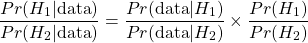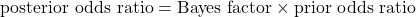Let us define ‘evidence‘ as the logarithm of the Bayes factor. The logarithmic scale is much more convenient to work with, as we will quickly see.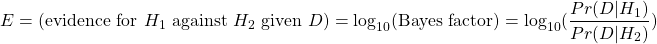Evidence is a quantity that depends on a particular observation or outcome and relates two hypothesis to one another. It can be positive or negative. For example, one can say Alice’s experimental results provide 3 units of evidence in favor of hypothesis H1 against hypothesis H2, or equivalently, -3 units of evidence in favor of hypothesis H2 against hypothesis H1.Figure 1: In this example, two hypotheses are being tested against one another. H1 is the hypothesis that the opossum is genetically closer to humpback whales than salmon is. H2 is the hypothesis that salmon is closer to humpback whales than the opossum. The data D that is being used to compare these hypotheses can be some DNA sequencing data, for instance. (The data here isn’t real).

But what does, for instance, 3 units of evidence mean? How do we interpret this number? 3 units of evidence means that it was 103=1000 times more likely to observe that particular outcome under hypothesis H1 compared to H2. And this number can be multiplied into one’s prior odds ratio to get a posterior odds ratio. If prior to seeing Alice’s data, you believed the probabiliy for H1 was half that of H2 (Pr(H1)/Pr(H2) = 0.5) then after seeing Alice’s data with 3 units of evidence, you update your probability odds ratio to Pr(H1)/Pr(H2) = 0.5×103 = 500. After seeing Alice’s data you attribute a probability to H1 that is 500 times larger than the probability you attribute to H2.

What’s nice about this definition is that evidence from independent observations can be added. This definition aligns with our colloquial usage of the term when we say “adding up” or “accumulating” evidence. So if Alice reports 3 units of evidence and Bob independently reports 2 units of evidence, it is as if we have a total of 5 units of evidence in favor H1 against H2. And if Carol then comes along with new experimental data providing 1.5 units of evidence in favor of H2 against H1 (conflicting with the other studies), the total resulting evidence is 3+2-1.5 = 3.5.

None of what I have written up to here is new. I am not even sure if my definition of evidence is entirely original. I’ve seen people use log likelihood ratios and call it evidence. But from here on is where we begin constructing something new.

It is commonly accepted that a scientific result needs to be replicated before it can be trusted. If two independent labs obtain congruent evidence for something (say Alice found 3 units of evidence and Bob found 2 units of evidence) it should count as stronger evidence than if just one of them found very strong evidence for it, (say Alice had instead found 5 units of evidence). But Bayes factors does not seem to reflect this very well since both cases are said to result in 5 units of evidence. To take this to an extreme, 10 independent studies all reporting 2 units of evidence in favor of H1 should prevail over one study reporting 20 units of evidence in favor of H2. But the way we currently set it up, they cancel each other out. How can we improve this framework to incorporate our intuition about the need for replication? I will discuss this in part 2.